## Calculate the wavelength of an electron mass 9.1*10^-31kg moving with a velocity of 2.0*10^6m/s (h=6.63*10^-34Js) (Hint: De Broglie’s wavel

Question

Calculate the wavelength of an electron mass 9.1*10^-31kg moving with a velocity of 2.0*10^6m/s (h=6.63*10^-34Js) (Hint: De Broglie’s wavelength)

in progress 0
2 months 2021-09-01T07:30:44+00:00 1 Answers 0 views 0

Wavelength,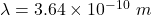Explanation:

We have,

Velocity an electron is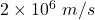Mass of an electron is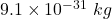It is required to find the wavelength of electron. The De-Broglie wavelength of an electron is given by :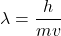h is Planck’s constant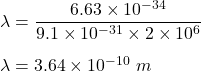So, the wavelength of an electron is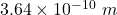.